SBAS572B May   2013  – March 2019

PRODUCTION DATA.

1. Features
2. Applications
3. Description
1.     Device Images
4. Revision History
5. Device Comparison Table
6. Pin Configuration and Functions
7. Specifications
8. Parameter Measurement Information
9. Detailed Description
1. 9.1 Overview
2. 9.2 Functional Block Diagram
3. 9.3 Feature Description
4. 9.4 Device Functional Modes
1. 9.4.1 CS Mode
2. 9.4.2 Daisy-Chain Mode
10. 10Application and Implementation
1. 10.1 Application Information
2. 10.2 Typical Applications
1. 10.2.1 DAQ Circuit for a 2.5-µs, Full-Scale Step Response
2. 10.2.2 DAQ Circuit for Lowest Distortion and Noise Performance at 400 kSPS
3. 10.2.3 Ultralow-Power DAQ Circuit at 10 kSPS
11. 11Power Supply Recommendations
12. 12Layout
13. 13Device and Documentation Support
14. 14Mechanical, Packaging, and Orderable Information

• DGS|10
• DRC|10
• DRC|10

### 7.9 Typical Characteristics

at TA = 25°C, AVDD = 3 V, DVDD = 3 V, VREF = 5 V, and fSAMPLE = 400 kSPS (unless otherwise noted)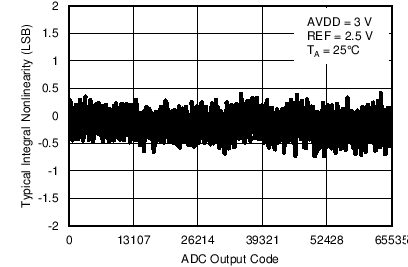Figure 4. Typical INL (VREF = 2.5 V)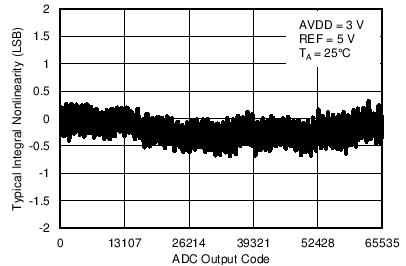Figure 6. Typical INL (VREF = 5 V)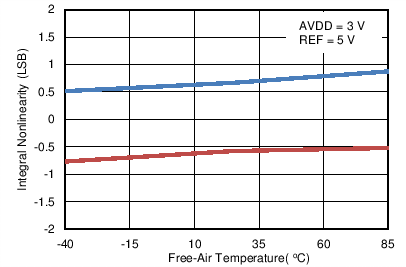Figure 8. INL vs Temperature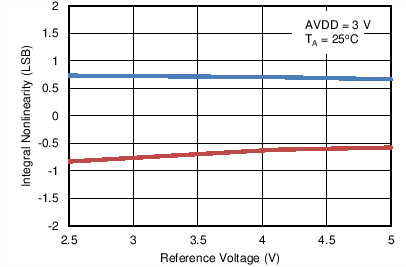Figure 10. INL vs Reference Voltage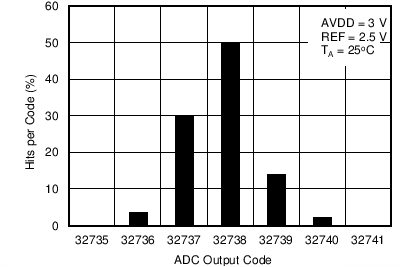Figure 12. DC Input Histogram (VREF = 2.5 V)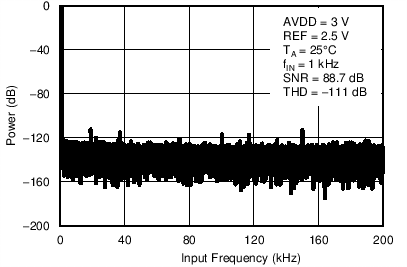Figure 14. Typical FFT (VREF = 2.5 V)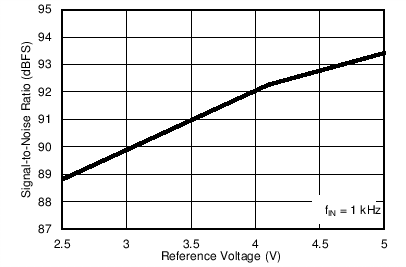Figure 16. SNR vs Reference Voltage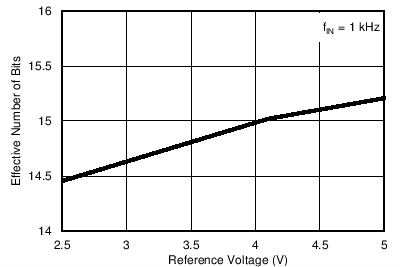Figure 18. ENOB vs Reference VoltageFigure 20. SFDR vs Reference Voltage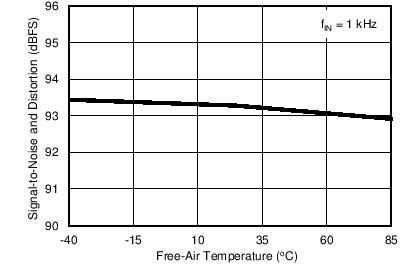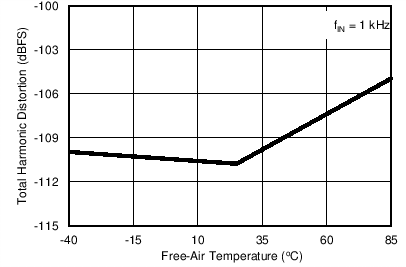Figure 24. THD vs Temperature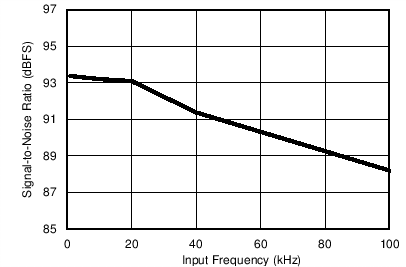Figure 26. SNR vs Input Frequency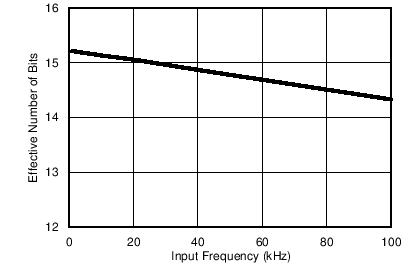Figure 28. ENOB vs Input Frequency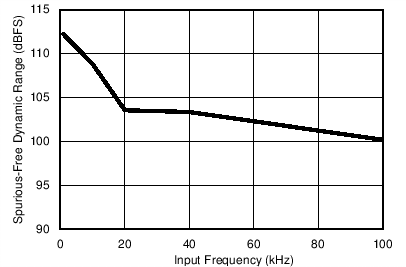Figure 30. SFDR vs Input Frequency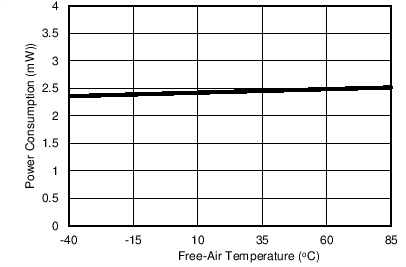Figure 32. Power Consumption vs TemperatureFigure 34. Power Consumption vs Throughput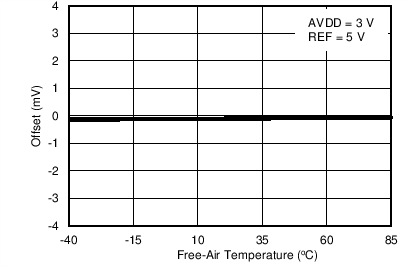Figure 36. Offset vs Temperature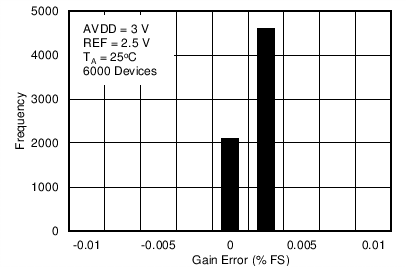Figure 38. Typical Distribution of Gain Error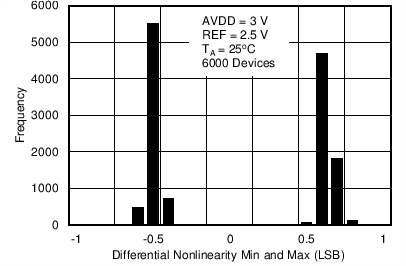Figure 40. Typical Distribution of Differential Nonlinearity (Minimum and Maximum)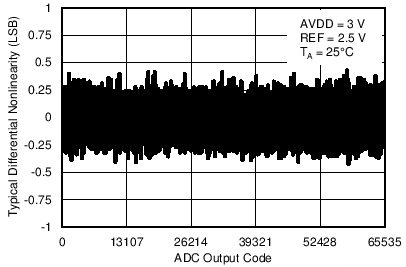Figure 5. Typical DNL (VREF = 2.5 V)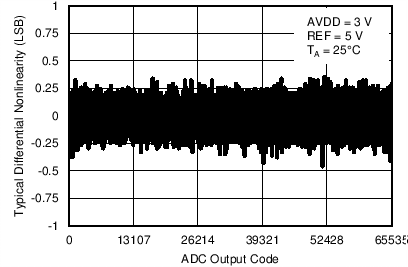Figure 7. Typical DNL (VREF = 5 V)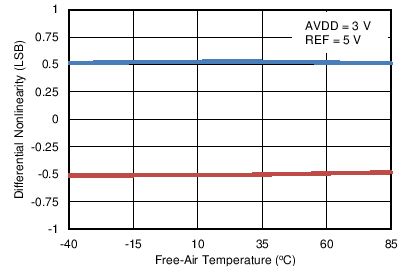Figure 9. DNL vs Temperature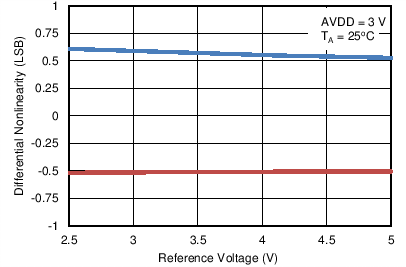Figure 11. DNL vs Reference Voltage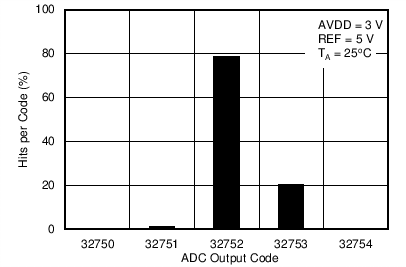Figure 13. DC Input Histogram (VREF = 5 V)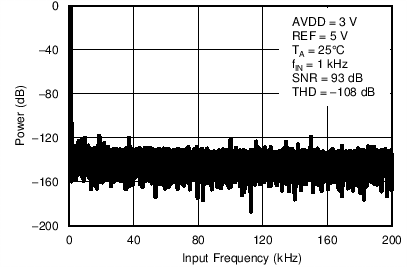Figure 15. Typical FFT (VREF = 5 V)Figure 17. SINAD vs Reference Voltage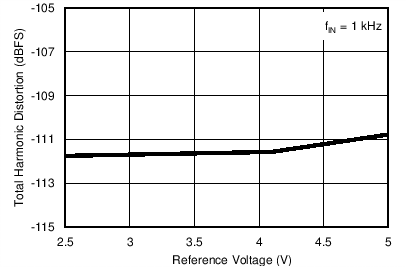Figure 19. THD vs Reference Voltage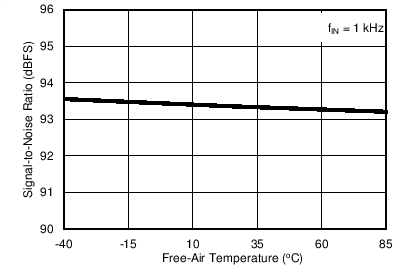Figure 21. SNR vs Temperature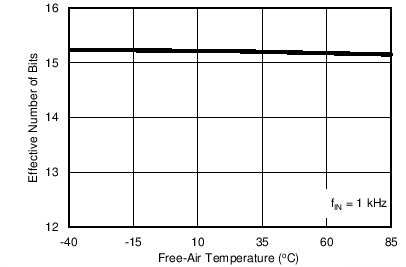Figure 23. ENOB vs Temperature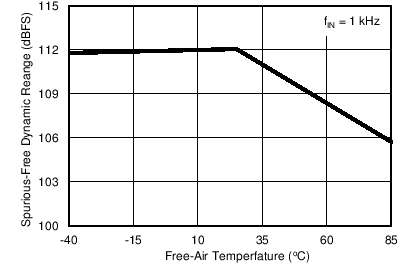Figure 25. SFDR vs Temperature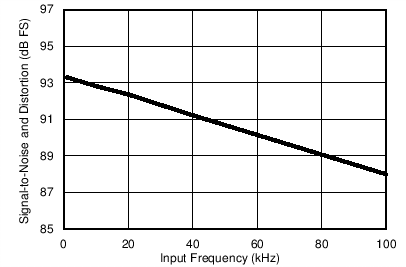Figure 27. SINAD vs Input Frequency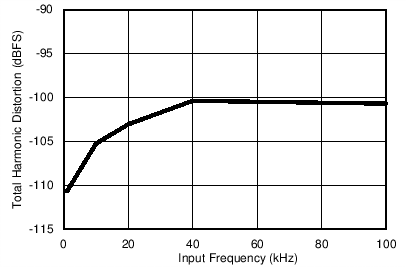Figure 29. THD vs Input Frequency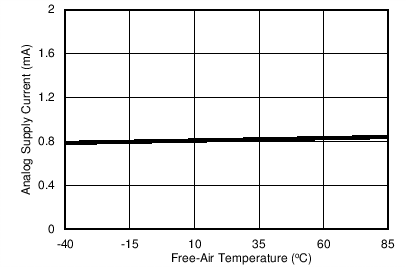Figure 31. Supply Current vs Temperature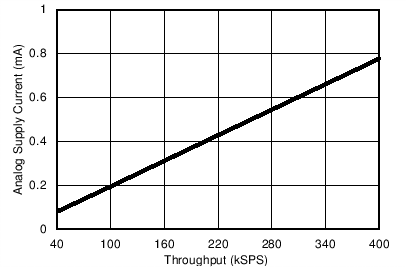Figure 33. Supply Current vs Throughput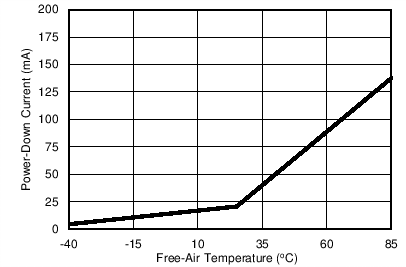Figure 35. Power-Down Current vs Temperature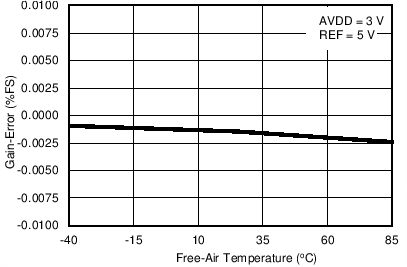Figure 37. Gain Error vs Temperature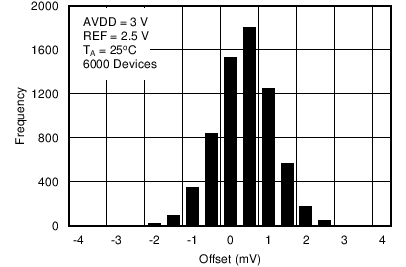Figure 39. Typical Distribution of Offset Error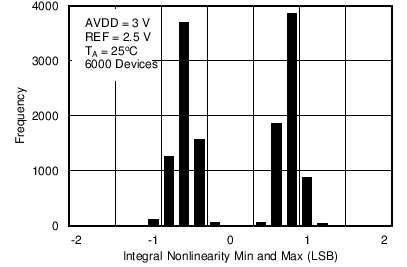Figure 41. Typical Distribution of Integral
Nonlinearity (Minimum and Maximum)